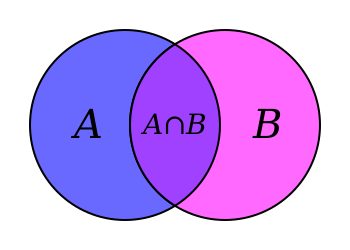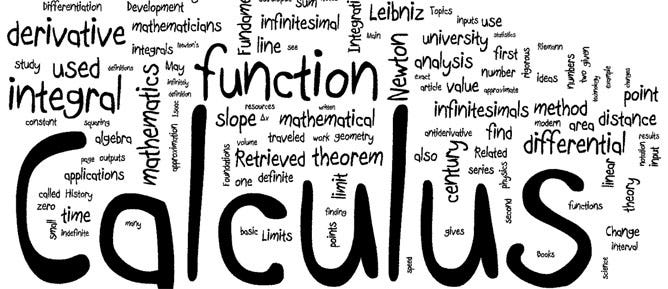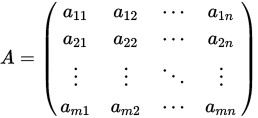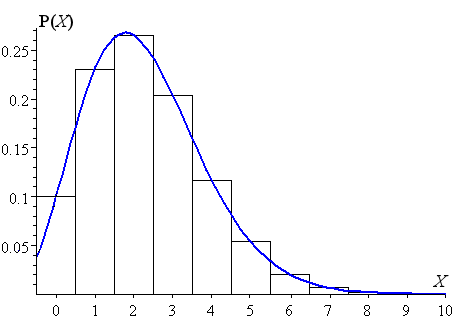# Introduction

## Do I need a strong math background to pursue a career as a data scientist?

We see a lot of questions like this. Its hard when you're trying to break into the field to know exactly how much math & stats you need. And, part of the reason for that is that it really depends.

Firstly, it depends on how a company is defining "data scientist." Some companies say "data scientist" but really mean "data engineer", which is much more focused on the software engineering side of things and strong with coding production systems, data storage and extraction, cluster management etc. The latter is less Math/Stats and more CS focused.

Secondly it depends on how a company is dividing responsibilities. Some look for people who are either strong in programming or strong in mathematics/statistics, and then combine them in a team. Others look for "fully fledged" data scientists who have the the deep insight in different models and when to apply which algorithms and can do all the implementation of the data. How the role you're looking at fits into these descriptions will effect how much math/stats you need to demonstrate.

Given the variance, the trick is to carefully disect the job posting and dig into the background of the current team. LinkedIn is a great place to do this. You can generally figure out the different roles (job titles) as well as see the skills/background people in these roles have.

That said, there are a few mainstays that, irrespective of role, you should be demonstrating on your resume. Either through your academic courses/coursework, online courses you've taken, or project work you've completed (including write-ups that demonstrate your understanding).

## Main skills

1. ### Basic algebraAlways a good idea to start at the root. Edifice of modern mathematics is built upon some key foundations — set theory, functional analysis, number theory etc. From an applied mathematics learning point of view, we can simplify studying these topics through some concise modules (in no particular order):

• set theory basics
• real and complex numbers and basic properties
• polynomial functions, exponential, logarithms, trigonometric identities
• inequalities, infinite series, binomial theorem
• graphing and plotting, Cartesian and polar co-ordinate systems, conic sections
• basic geometry and theorems, triangle properties
2. ### CalculusSir Issac Newton wanted to explain the behavior of heavenly bodies. But he did not have a good enough mathematical tool to describe his physical concepts. So he invented this (or a certain modern form) branch of mathematics when he was hiding away on his countryside farm from the plague outbreak in urban England. Since then, it is considered the gateway to advanced learning in any analytical study — pure or applied science, engineering, social science, economics, ...

Not surprisingly then, the concept and application of calculus pops up in numerous places in the field of data science or machine learning. Most essential topics to be covered are as follows:

• functions of single variable, limit, continuity and differentiability
• mean value theorems, indeterminate forms and L’Hospital rule
• maxima and minima
• product and chain rule
• Taylor’s series
• fundamental and mean value-theorems of integral calculus
• evaluation of definite and improper integrals, h) Beta and Gamma functions
• functions of two variables, limit, continuity, partial derivatives
• basics of ordinary and partial differential equations
3. ### Linear AlgebraGot a new friend suggestion on Facebook? A long lost professional contact suddenly added you on LinkedIn? Amazon suddenly recommended an awesome romance-thriller for your next vacation reading? Or Netflix dug up for you that little-known gem of a documentary which just suits your taste and mood?

Doesn’t it feel good to know that if you learn basics of linear algebra, then you are empowered with the knowledge about the basic mathematical object that is at the heart of all these exploits by the high and mighty of the tech industry?

At least, you will know the basic properties of the mathematical structure that controls what you shop on Target, how you drive using Google Map, which song you listen to on Pandora, or whose room you rent on Airbnb.

The essential topics to study are (not an ordered or exhaustive list by any means):

• basic properties of matrix and vectors —scalar multiplication, linear transformation, transpose, conjugate, rank, determinant,
• inner and outer products
• matrix multiplication rule and various algorithms
• matrix inverse
• special matrices — square matrix, identity matrix, triangular matrix, idea about sparse and dense matrix, unit vectors, symmetric matrix, Hermitian, skew-Hermitian and unitary matrices
• matrix factorization concept/LU decomposition, Gaussian/Gauss-Jordan elimination, solving Ax=b linear system of equation
• vector space, basis, span, orthogonality, orthonormality, linear least square, h) singular value decomposition
• eigenvalues, eigenvectors, and diagonalization
4. ### Statistics and Probability

Only death and taxes are certain, and for everything else there is normal distribution.The importance of having a solid grasp over essential concepts of statistics and probability cannot be overstated in a discussion about data science. Many practitioners in the field actually call machine learning nothing but statistical learning. I followed the widely known “An Introduction to Statistical Learning” while working on my first MOOC in machine learning and immediately realized the conceptual gaps I had in the subject. To plug those gaps, I started taking other MOOCs focused on basic statistics and probability and reading up/watching videos on related topics. The subject is vast and endless, and therefore focused planning is critical to cover most essential concepts. I am trying to list them as best as I can but I fear this is the area where I will fall short by most amount.

• data summaries and descriptive statistics, central tendency, variance, covariance, correlation
• Probability: basic idea, expectation, probability calculus, Bayes theorem, conditional probability
• probability distribution functions — uniform
• normal, binomial, chi-square, student’s t-distribution, central limit theorem
• sampling, measurement, error, random numbers
• hypothesis testing, A/B testing, confidence intervals, p-values
• ANOVA
• linear regression
• power, effect size, testing means
• research studies and design-of-experiment
Page structure
Terms